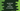# 4 different JavaScript program to find the factorial of a number## JavaScript program to find the factorial of a number:

This post will show you how to find the factorial of a number in JavaScript. We will learn different ways to solve this problem. The program will take one number as input from the user and it will print the factorial on the console.

The factorial of a number is equal to the multiplication of all numbers from 1 to that number. For example, if the number is 5, the factorial of 5 is 54321 = 120.

! is used as the symbol of factorial. So,

``Factorial of n, n! = 1 * 2 * 3 *......*n``

Factorial of 0 is 1.

### Method 1: JavaScript program to find the factorial of a number by using a for loop:

The below program uses a for loop to find the factorial of a given number:

``````function getFactorial(n) {
if (n < 0) {
return;
}

let factorial = 1;

for (let i = 2; i <= n; i++) {
factorial *= i;
}

return factorial;
}

const numbers = [-1, 0, 1, 5, 8, 10];

for (const n of numbers) {
console.log(`\${n}! = \${getFactorial(n)}`);
}``````

In this program,

• getFactorial is a function that takes one number as its parameter and returns the factorial of the number.
• If the number is less than 0, it returns from the function.
• Else, it creates a variable factorial and assigns it 1. Then it runs one for loop from i = 2 to n and multiplies the i with factorial.
• Once the loop ends, the factorial variable will hold the final factorial. It returns this value. If the number is 1 or 0, it will not enter the for loop and 1 will be returned.
• This program is finding the factorial of all the values of the array numbers.

If you run this program, it will print the below output:

``````-1! = undefined
0! = 1
1! = 1
5! = 120
8! = 40320
10! = 3628800``````

### Method 2: JavaScript program to find the factorial of a number by using a while loop:

We can replace the for loop with a while loop in the above example. It will work in a similar way.

``````function getFactorial(n) {
if (n < 0) {
return;
}

let factorial = 1;
let i = 2;

while (i <= n) {
factorial *= i;
i++;
}

return factorial;
}

const numbers = [-1, 0, 1, 5, 8, 10];

for (const n of numbers) {
console.log(`\${n}! = \${getFactorial(n)}`);
}``````

The variable i is initialized before the loop starts and at the end of each iteration, it is incremented by 1. If you run this program, it will give a similar output.

### Method 3: Recursively find the factorial of a number in JavaScript:

We can also write a recursive function to find the factorial. A recursive function calls itself again and again to find a solution. To find the factorial recursively, we have to call the same function with a value decremented by 1 on each call. It will stop once the value becomes 1.

Below is the complete program in JavaScript:

``````function getFactorial(n, fact) {
if (n < 0) {
return;
}

if (n <= 1) {
return fact;
}

return getFactorial(n - 1, fact * n);
}

const numbers = [-1, 0, 1, 5, 8, 10];

for (const n of numbers) {
console.log(`\${n}! = \${getFactorial(n, 1)}`);
}``````
• The getFactorial function is changed to a recursive function. It takes the number and also the value of the current factorial as its parameters. The factorial value, fact is passed as 1 while calling this function.
• If the value of n is less than 0, it returns from the function.
• If the value of n is less than or equal to 1, it returns the factorial value.
• Else, it calls the same function getFactorial and returns the result. On each recursive call, it decrements the value of n by 1 and multiplies the factorial value with the current value of n. The recursive call stops once the value of n becomes 1.

If you run this program, it will print the same output.

### Method 4: Recursive Arrow function to find the factorial:

We can remove the second parameter to pass to the recursive function. Instead, the number can be multiplied with the recursive result. The below program uses an arrow function to find the result recursively:

``````const getFactorial = (n) => {
if (n < 0) {
return;
}

return n > 1 ? n * getFactorial(n - 1) : 1;
};

const numbers = [-1, 0, 1, 5, 8, 10];

for (const n of numbers) {
console.log(`\${n}! = \${getFactorial(n)}`);
}``````

This is a better approach than the previous example. It is taking only one parameter, the number to find the factorial and based on its value, it calculates the factorial.

It will print the same output:

``````-1! = undefined
0! = 1
1! = 1
5! = 120
8! = 40320
10! = 3628800``````